# Raw WebGL

Nick Desaulniers

## Warning

Graphics programming is a rabbit hole that is infinitely deep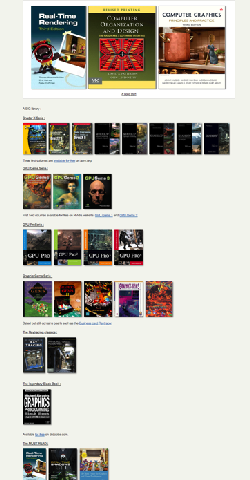## Warning

Graphics programming is equal parts physics (how do I accurately model the physical phenomina that I am seeing) and computer science (how do I calculate all this math and still hit 16.66ms).

It's all that math you learned but never had a chance to apply.

• Geometry
• Trigonometry
• Linear Algebra

## History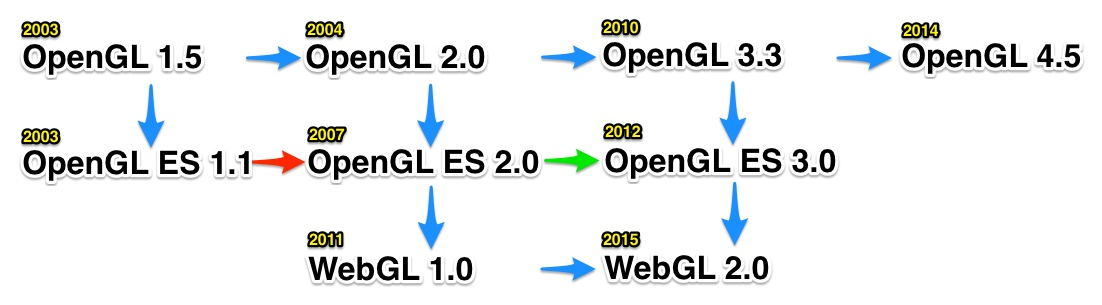## Pipeline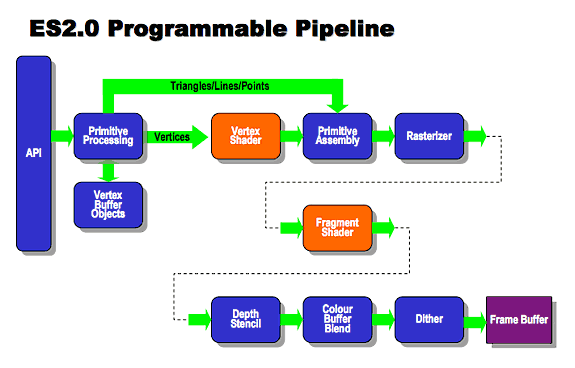## Application?All of these are optional except a single shader pair

## Vertex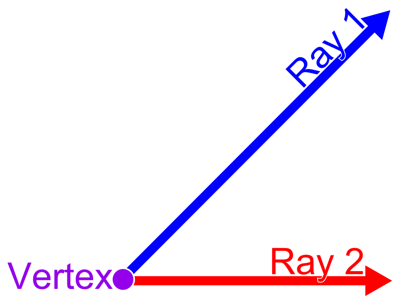A special kind of point that describes the corners or intersections of geometric shapes.

## Polygon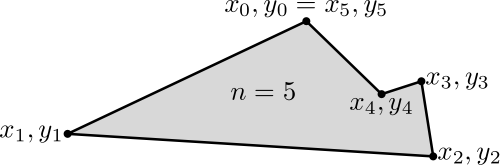A figure bounded by edges, and the points where two edges meet are the polygon's vertices.

## Mesh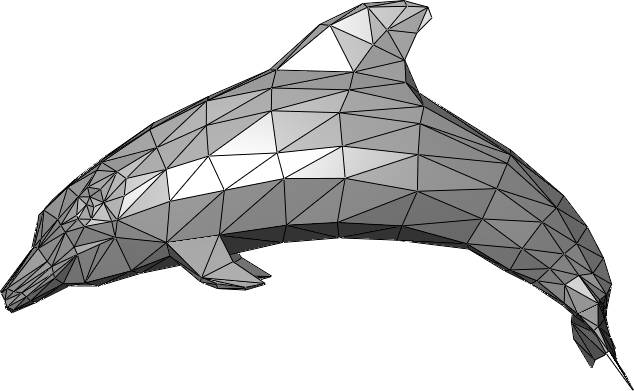A polygon mesh is a collection of vertices, edges and faces that defines the shape of a polyhedral object in 3D computer graphics and solid modeling. AKA Geometric Model.

## Triangles

Why do we use triangles, as opposed to squares (aka quads)?

The vertices of a triangle are guaranteed to lie along the same plane.

## Buffer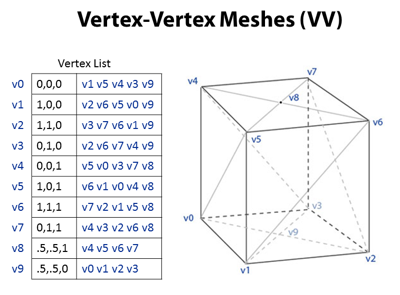A data buffer is a region of a memory used to temporarily store data while it is being moved from one place to another.

## Affine Transformation

A function between affine spaces which preserves points, straight lines, and planes. Parallel lines stay parrallel. Doesn't preserve angles or distances, but does for ratios between points lying on a straight line.

## Translate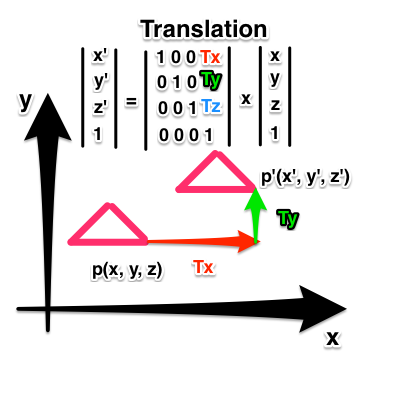## Rotate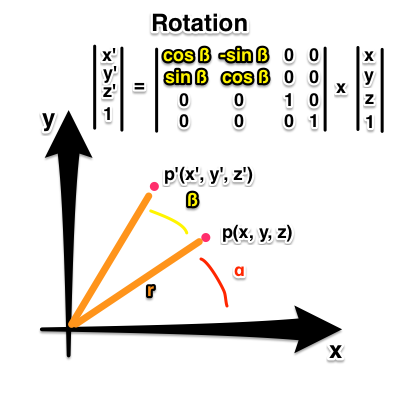## Scale## Homogenous Coordinates

p(x, y, z, w) allows us to:

Represent infinite distances as (x/w, y/w, z/w) as w -> 0 which allows us to do perspective transformations.

Compose transformations as a series of matrix multiplications.

## Perspective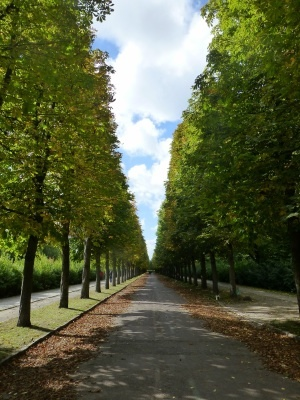Does the right side of the road eventually touch the left side?

Are the trees getting shorter?

## Frustum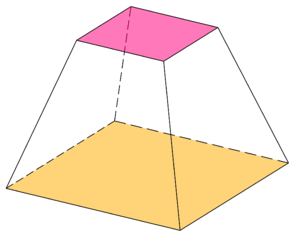The portion of a solid (normally a cone or pyramid) that lies between two parallel planes cutting it.

## Viewing Frustum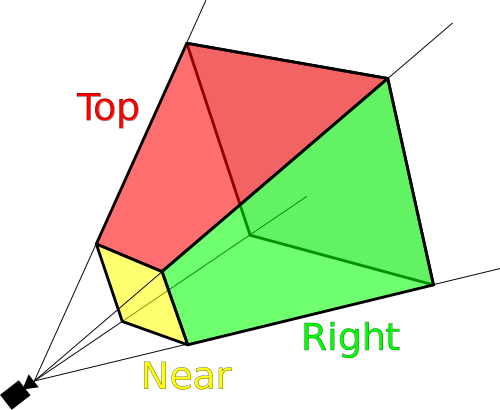AKA Field of View

## Bitmap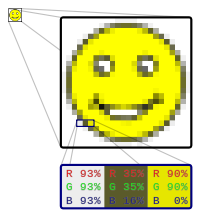A data structure representing a rectangular grid of pixels, or points of color, viewable via a display medium.

## Textures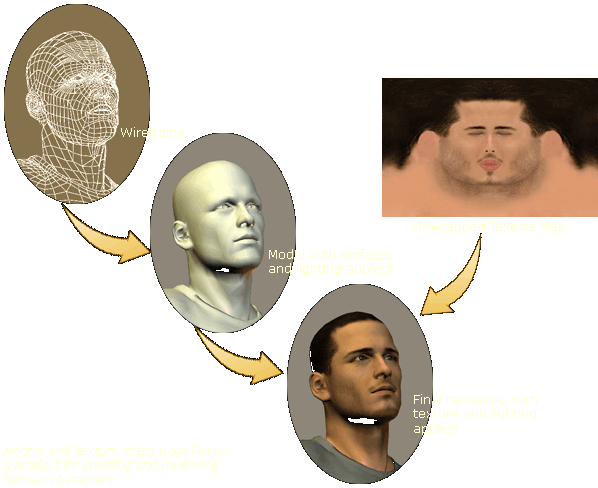A bitmap that will later be applied or mapped onto a mesh or model.

## SamplingThe reduction of a continuous signal to a discrete signal.

Allows us to quickly approximate an integral.

## Rasterization

The process to convert shapes, defined in a vector format into fragments (pixels or dots) for display on a video screen.

## Fragment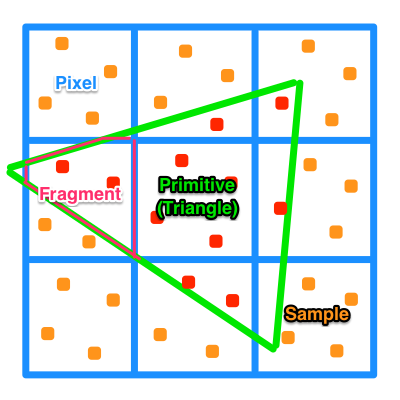The pixel generated by the rasterization process process which has color, depth, value, texture coordinates, and more.

## Frame

One of the many still images which compose the complete moving picture; each image looks rather like a framed picture when examined individually.

## Real Time Rendering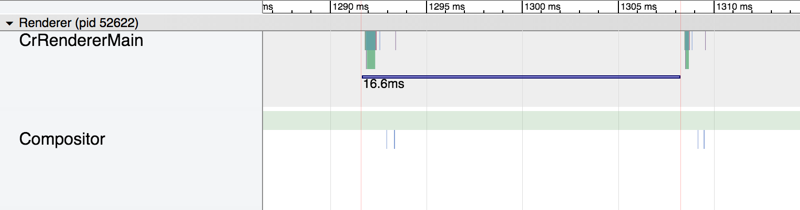Doing the rendering computation fast enough, so that the series of rendered images allow for interaction taking into account user input.

• 30 fps = 33.3 ms
• 60 fps = 16.6 ms
• 90 fps = 11.1 ms

## Pre Rendering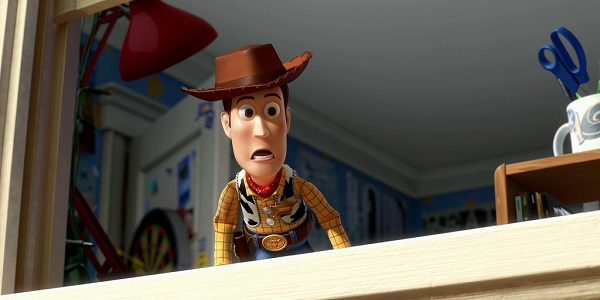Opposite of real time rendering.

Toy Story 3 took on average 7 hours to render 1 frame (24 fps), and at most 39 hours.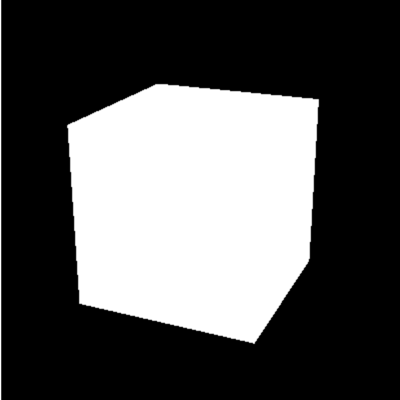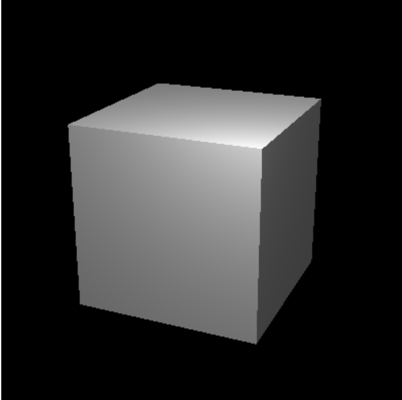Depicting depth perception in 3D models by varying levels of darkness.

## Material

A shader program that implements a scattering function for a set of polygons.

Small programs that run massively parallel on the GPU.

Shaders come in pairs (for now) of vertex and fragment.

Shaders can be mixed and matched if the have the same output to input.

Vertex shaders are run for each vertex.

Fragment shaders are run for each fragment, with color values being interpolated inbetween.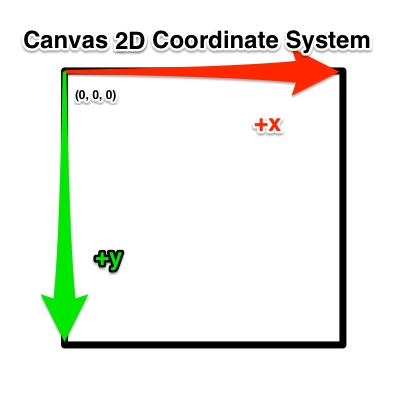Also, the DOM.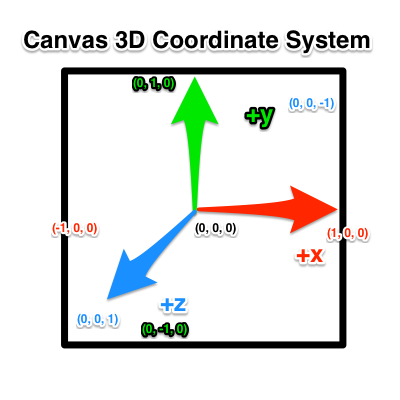Anything outside of the 2x2x2 box is culled (doesn't get drawn).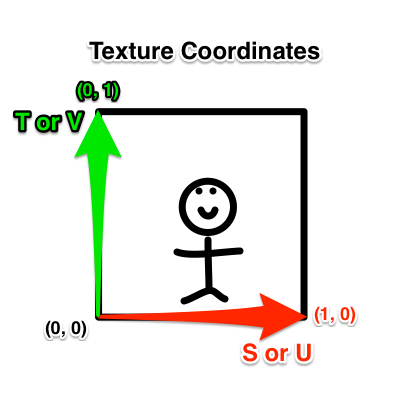For all sizes of images, (1, 1) represents top right.

Most bitmaps store the inverse vertical axis.

## The Canvas

``````

Oops, your browser doesn't support html5 canvas!

``````

## Getting a WebGL Context

``````
var canvas = document.getElementById('my_canvas');
var gl = canvas.getContext('webgl') ||
canvas.getContext('experimental-webgl');
if (!gl) throw new Error('browser may not support webgl');
``````

## Clearing the Canvas

``````
gl.clearColor(0.0, 0.0, 0.0, 1.0);
gl.clear(gl.COLOR_BUFFER_BIT);
``````
Example

``````
attribute vec4 aPosition;
void main () {
gl_Position = aPosition;
gl_PointSize = 10.0;
}
``````

``````
precision mediump float;
uniform vec4 uFragColor;
void main () {
gl_FragColor = uFragColor;
}
``````

Written in GLSL

## Attributes and Uniforms

Uniforms and Attributes are inputs to shaders.

Uniforms are the same (uniform) for all vertices.

Attributes are unique per vertex.

Attributes are only inputs to vertex shaders.

``````
function compile (gl, type, shaderSrc) {

}

};
``````

``````
var program = gl.createProgram();

throw new Error(gl.getProgramInfoLog(program));
}

return program;
};
gl.useProgram(program);
``````

A 3D application may use more than one gl program.

## Uniforms and Attributes

``````
var aPosition = gl.getAttribLocation(program, 'aPosition');
var uFragColor = gl.getUniformLocation(program, 'uFragColor');
``````

## Attributes Directly

``````
gl.vertexAttrib2f(aPosition, 0.0, 0.0);
gl.uniform4f(uFragColor, 1.0, 0.0, 0.0, 1.0);
``````

## Draw

``````
// takes a mode, first, count
gl.drawArrays(gl.POINTS, 0, 1);
``````
Example

## Separation of Concerns

Move shaders from HTML or JS into their own files, load with XHR.

Though, we can do cool tricks by procedurally generating strings of shaders at runtime.

## Reflection

We can use reflection of a gl program to get references to its attributes and uniforms. This step is not strictly necessary, but can help you get references when shaders have many inputs.

``````
var numAttributes = gl.getProgramParameter(program, gl.ACTIVE_ATTRIBUTES);
for (var i = 0; i < numAttributes; ++i) {
gl.getAttribLocation(program, gl.getActiveAttrib(program, i).name);
}

var numUniforms = gl.getProgramParameter(program, gl.ACTIVE_UNIFORMS);
for (i = 0; i < numUniforms; ++i) {
gl.getUniformLocation(program, gl.getActiveUniform(program, i).name);
}
``````

## Modularize and Reuse

Move compile, link, reflection, and other helper functions into reusable file.

Example

## Drawing Points

First, we need to beef up our shaders.

``````
attribute vec4 aPosition;
attribute float aPointSize;
attribute vec4 aColor;
varying vec4 vColor;

void main () {
gl_Position = aPosition;
gl_PointSize = aPointSize;
vColor = aColor;
}
``````
``````
precision mediump float;
varying vec4 vColor;

void main () {
gl_FragColor = vColor;
}
``````

## Multiple Draw Calls

As a naive first attempt, let's draw each point with its own draw call.

``````
var p = [
{ x:  0.0, y:  0.5, sz: 10.0, r: 1.0, g: 0.0, b: 0.0 },
{ x: -0.5, y: -0.5, sz: 20.0, r: 0.0, g: 1.0, b: 0.0 },
{ x:  0.5, y: -0.5, sz: 30.0, r: 0.0, g: 0.0, b: 1.0 }
];
for (var i = 0; i < p.length; ++i) {
gl.vertexAttrib4f(attributes.aPosition, p[i].x, p[i].y, 0.0, 1.0);
gl.vertexAttrib1f(attributes.aPointSize, p[i].sz);
gl.vertexAttrib4f(attributes.aColor, p[i].r, p[i].g, p[i].b, 1.0);
gl.drawArrays(gl.POINTS, 0, 1);
}
``````
Example

## Multiple Draw Calls

Multiple draw calls sometimes are needed to build up a scene.

Draw calls can be the most expensive call.

Try to minimize draw calls.

## Multiple Buffers

Instead, we can push data in multiple buffers to the GPU.

``````
function initBuffer (gl, data, elemPerVertex, attribute) {
var buffer = gl.createBuffer();
if (!buffer) throw new Error('Failed to create buffer.');
gl.bindBuffer(gl.ARRAY_BUFFER, buffer);
gl.bufferData(gl.ARRAY_BUFFER, data, gl.STATIC_DRAW);
gl.vertexAttribPointer(attribute, elemPerVertex, gl.FLOAT, false, 0, 0);
gl.enableVertexAttribArray(attribute);
};
var positions = new Float32Array([0.0, 0.5, -0.5, -0.5, 0.5, -0.5]);
var pointSize = new Float32Array([10.0, 20.0, 30.0]);
var colors = new Float32Array([1.0, 0.0, 0.0, 0.0, 1.0, 0.0, 0.0, 0.0, 1.0]);
initBuffer(gl, positions, 2, attributes.aPosition);
initBuffer(gl, pointSize, 1, attributes.aPointSize);
initBuffer(gl, colors, 3, attributes.aColor);
``````
Example

## Warning

Copying memory (uploading buffers) from the main memory to the video memory can be expensive. It can be faster to upload a single larger buffer in one go than multiple smaller buffers individually.## Interleaved Buffer

``````
var data = new Float32Array([
0.0,  0.5,   10.0,   1.0, 0.0, 0.0,
-0.5, -0.5,   20.0,   0.0, 1.0, 0.0,
0.5, -0.5,   30.0,   0.0, 0.0, 1.0
]);
var bpe = data.BYTES_PER_ELEMENT;
var buffer = gl.createBuffer();
if (!buffer) throw new Error('Failed to create buffer.');
gl.bindBuffer(gl.ARRAY_BUFFER, buffer);
gl.bufferData(gl.ARRAY_BUFFER, data, gl.STATIC_DRAW);
gl.vertexAttribPointer(attributes.aPosition, 2, gl.FLOAT, false, 6 * bpe, 0);
gl.enableVertexAttribArray(attributes.aPosition);
gl.vertexAttribPointer(attributes.aPointSize, 1, gl.FLOAT, false, 6 * bpe, 2 * bpe);
gl.enableVertexAttribArray(attributes.aPointSize);
gl.vertexAttribPointer(attributes.aColor, 3, gl.FLOAT, false, 6 * bpe, 3 * bpe);
gl.enableVertexAttribPointer(attributes.aColor);
``````
Example

## Warning

What if only some of the data in our buffer is changing every frame of animation?

It might be wiser to use multiple buffers instead of interleaved.

There is no one right answer; depends on situation.

Profile your app and figure out what works best.

Other tricks like Element Array Buffers can help reduce memory usage.

## Drawing Modes

gl.drawArrays(mode, start, numVertices) can take:

• gl.POINTS
• gl.LINES
• gl.LINE_STRIP
• gl.LINE_LOOP
• gl.TRIANGLES
• gl.TRIANGLE_STRIP
• gl.TRIANGLE_FAN

Example

## Perspective

In order to properly draw a 3D scene in perspective we need 3 things:

1. Matrix of our model's transformations relative to its original coords. (Model Matrix)

2. Matrix of where we are relative to model, where we are looking, and the up direction. (View Matrix)

3. Matrix decribing viewing angle, aspect ratio, and near and far clipping plane of our viewing frustum. (Projection Matrix)

Examples

## Animation

``````
function animate (time) {
// update
mat4.rotateY(modelMatrix, modelMatrix, Math.PI / 180);
gl.uniformMatrix4fv(modelUniform, false, modelMatrix);

// render
gl.clear(gl.COLOR_BUFFER_BIT | gl.DEPTH_BUFFER_BIT);
gl.drawArrays(gl.TRIANGLES, 0, n);
requestAnimationFrame(animate);
};
``````
Example

## Textures

#### Initialization

``````
var texture = gl.createTexture();
gl.activeTexture(gl.TEXTURE0);
gl.bindTexture(gl.TEXTURE_2D, texture);
gl.texParameteri(gl.TEXTURE_2D, gl.TEXTURE_MIN_FILTER, gl.LINEAR);
gl.texParameteri(gl.TEXTURE_2D, gl.TEXTURE_MAG_FILTER, gl.LINEAR);
gl.texParameteri(gl.TEXTURE_2D, gl.TEXTURE_WRAP_S, gl.CLAMP_TO_EDGE);
gl.texParameteri(gl.TEXTURE_2D, gl.TEXTURE_WRAP_T, gl.CLAMP_TO_EDGE);
gl.uniform1i(uSampler, 0);
``````

Multiple textures can use the same texture unit, but shaders can only refer to the latest bound texture.

## Textures

#### Sampling

``````
gl.bindTexture(gl.TEXTURE_2D, texture);
gl.pixelStorei(gl.UNPACK_FLIP_Y_WEBGL, 1);
gl.texImage2D(gl.TEXTURE_2D, 0, gl.RGBA, gl.RGBA, gl.UNSIGNED_BYTE,
imgArrayBufferViewVideoOrCanvas);
``````

Can be done during initialization for static images.

Must be done during requestAnimationFrame loop for video.

Must be done when limited on texture units per draw call.

Example

## Warning

Try to minimize texture swapping between frames.

#### Illumination Models

Lambert - The shade is the cosine of the angle between surface normal and light vector.

Blinn-Phong - The shade is composed of a specular, diffuse, ambient, and shininess components of the material.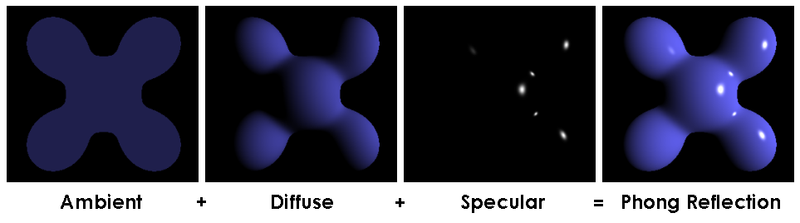#### Interpolation Techniques

Flat shading - Each polygon has its own color - Disco Ball

Gouraud shading - Colors calculated per vertex - Mach Bands

Phong shading - Colors calculated per pixel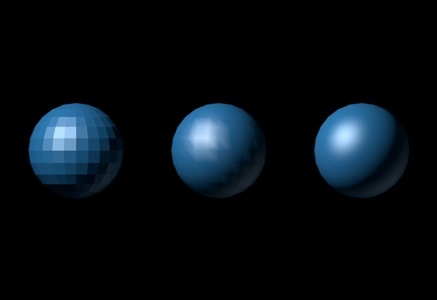All of this can be done with what you now know.

Light directions and material reflective coefficients are buffered.

1. Making WebGL Dance - Steve Wittens
2. WebGL 101 - Eric Möller
3. WebGL Programming Guide - Matsuda, Lea
5. webgl-2d source
6. Computer Graphics: Principles and Practice
7. Three.js source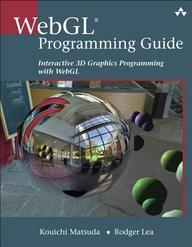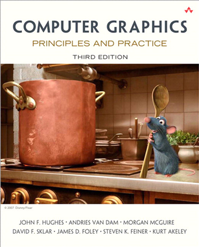## ThanksNick Desaulniers